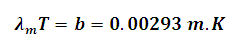# Wein's Displacement Law Calculator

This Calctown Calculator calculates the wavelength of energy emitted by a black body using Wein's Displacement Law.

K

#### Result

μmwhere

λm = wavelength for maximum emitted energy

T = temperature of the body

b = constant = 0.00293 m.K